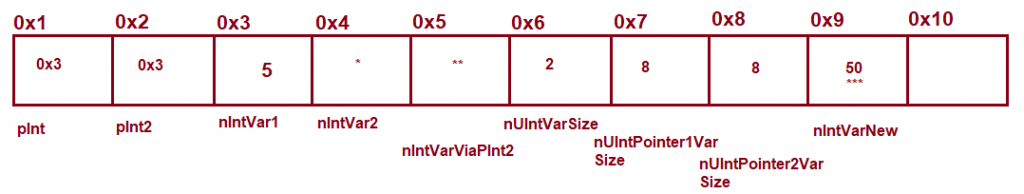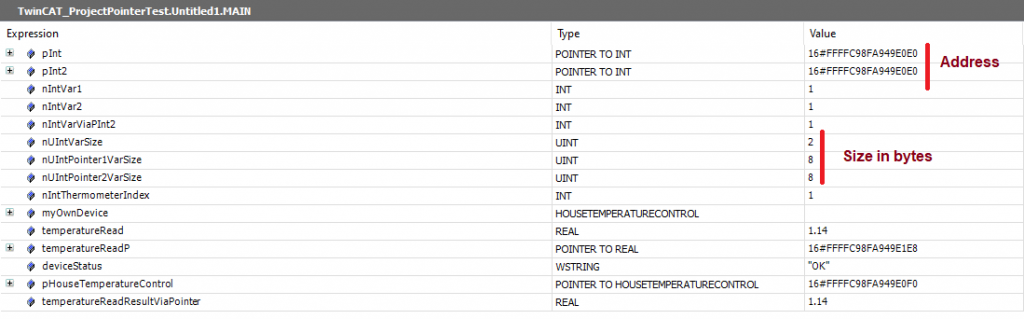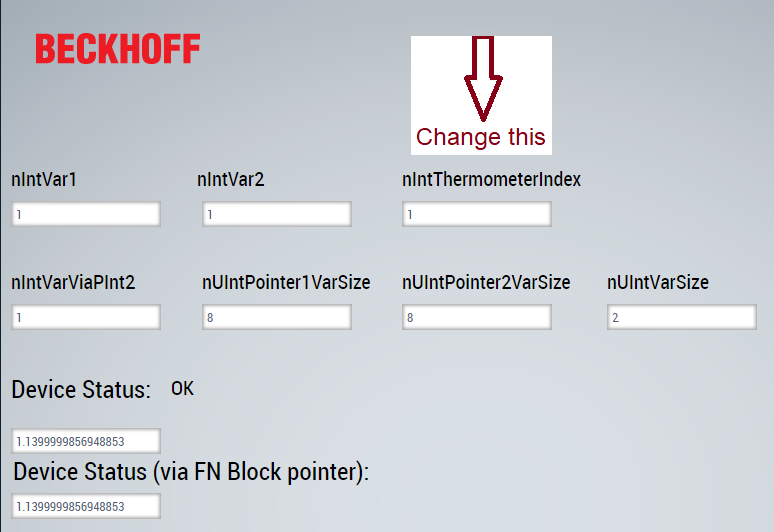## Pointer and Memory Management

Pointer :

Pointers store the addresses of variables, function blocks and programs, while an application program is running. A pointer points to one of the named objects or to a variable with any data type.

Dereferencing a pointer means obtaining the value of the address to which the pointer points. You can dereference a pointer by appending the content operator to the pointer identifier, for example, see pInt^ in the example below.

You can use the address operator ADR to assign the address of a variable to a pointer.

An analogy to pointer:

Say, for example, we have a row of lockers (memory for computer), there is a person who manages the lockers, I tell him to save my 5 € in the locker. I don’t know where he will save it but he knows.

`nIntVar1 : INT := 5;  // I tell him to save somewhere.`

If I know where he has saved it, I can take the money directly.

`pInt := ADR(nIntVar1); //pInt is the address where it was saved`

I can pick the money directly by the following way

`nIntVar2 := pInt^; //Give me what has been stored at pInt place`

One important note is that we should have an initialization function where we do the initialization for a single time, we don’t have it in the sample so it is done in each plc cycle

Example:

`PROGRAM MAIN VAR //pointer to INT pInt : POINTER TO INT; pInt2 : POINTER TO INT; //declaration of variables nIntVar1 and nIntVar2 nIntVar1 : INT := 5; nIntVar2 : INT; nIntVarViaPInt2 : INT; //nUIntVarSize holds the size of INT in bytes nUIntVarSize : UINT; //nUIntPointer1VarSize holds the size of Pointer in bytes nUIntPointer1VarSize : UINT; //nUIntPointer2VarSize holds the size of Pointer in bytes nUIntPointer2VarSize : UINT; nIntThermometerIndex : INT := 50; //Pointer with Function block myOwnDevice : HOUSETEMPERATURECONTROL; temperatureRead : REAL := 0.0; temperatureReadP : POINTER TO REAL ; deviceStatus : WSTRING := "NOK"; //Pointer to function block pHouseTemperatureControl : POINTER TO HOUSETEMPERATURECONTROL; temperatureReadResultViaPointer : REAL := 0.0; END_VAR`
```//program Body//pointer pInt is assigned to address of nIntVar1

//PInt2 point to same location where pInt point
pInt2 := pInt;

//value 5 of nVar1 is assigned to variable nIntVar2 by dereferencing of pointer pSample
nIntVar2 := pInt^;

nIntVarViaPInt2 := pInt2^;

nUIntVarSize := SIZEOF(INT);
//both should be same
nUIntPointer1VarSize := SIZEOF(pInt);
nUIntPointer2VarSize := SIZEOF(pInt2);
pInt^ := nIntThermometerIndex;

//Pointer with Function block
myOwnDevice(deviceID:=pInt, deviceName := "My Device Name");
deviceStatus := "OK";
ELSE
deviceStatus := "NOK";
END_IF

pInt is called a pointer variable because it holds the address of another variable(nIntVar1). The address of a variable is assigned by the ADR operator. The operating system assigned the address for the variables.

We have declared another pointer variable and assigned it to the previous pointer variable. Now if we change the content of a pointer variable then others will be updated as well because both have the same address.

The pointer can be passed to another function block just like a variable. The pointer can be dereferenced to get the value it holds. We have to be careful when dereferencing it if it is a valid pointer, otherwise, we can have a blue screen.

If you change, nIntVarNew variable to something then you see that some other content will change, but if you change other variables then you notice that other variables are not changed, you can do research based on what we have described.

The following image shows some simplified memory layout of the PLC memory (actual addresses are different).

In this simplified example, we see pInt and pInt2 variable contains the address 0x3. This is also the address of the variablenIntVar1. If we assign something to the nIntVar1 and if some pointer variables point to this address that will contain the same data.

We can dereference in the following way.

`nIntVar2 := pInt^;`

By the above statement we say pInt is a pointer variable give me the content of the address it hold and assign it to nIntVar2.

In this case, nIntVar2 will be same as nIntVar1.*   Assign the content of address pInt  to nIntVar2

*   Assign the content of address pInt2  to nIntVarViaPInt2

***

`pInt^ := nIntVarNew;`

Assign the value of nIntVarNew to the address which is hold by pIntThe HMI for manipulating variables:One important note is that we should have an initialization function where we do the initialization for a single time, we don’t have it in the sample so it is done in each plc cycle

Down load the sample TwinCAT ProjectPointerTest.zip

##### Memcpy

Library contains a number of functions which provide direct access to memory areas in the PLC runtime system of the PLC Controller. Default editor includes the library by default.

The fact that these functions allow direct access to the physical memory of the PLC. Misuse of such functions can result in a system crash, or in access to forbidden memory areas. So we need to be careful when we are using these function.

MEMCMP Compares the values of variables in two memory areas

MEMCPY Copies the values of variables from one memory area to another

MEMSET Sets the variables in a memory area to a particular value

##### Memcpy example

//Declaration

PROGRAM MAIN

VAR

org : DINT :=12;

dest : DINT :=13;

another : DINT := 14;

myTimer : TON;

startTimer : BOOL := FALSE;

END_VAR

//Body

myTimer(IN:= startTimer, PT:=T#1S);

IF myTimer.Q = TRUE THEN

myTimer(IN:= FALSE);

org:= org +1;

END_IF